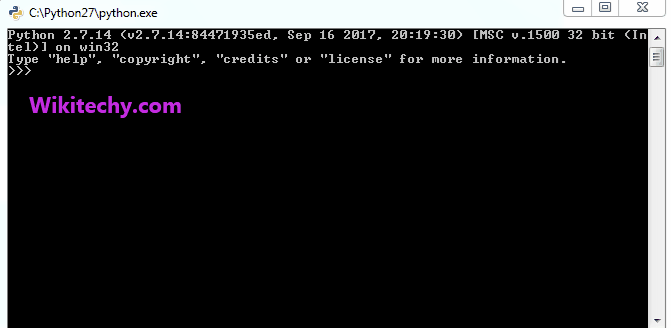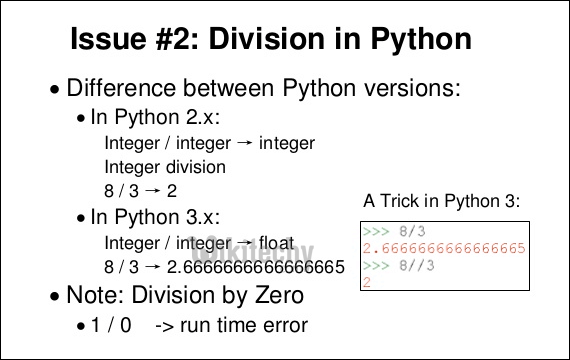# python tutorial - Division in Python | Division Operators in Python - learn python - python programmingLearn Python - Python tutorial - interesting-facts-about-strings-python divide operator - Python examples - Python programs

Consider below statements in Python 2.7

Output:

```2
-3
```First output is fine, but the second one may be surprising if we are coming Java/C++ world. In Python 2.7, the “/” operator works as a floor division for integer arguments. However, the operator / returns a float value if one of the arguments is a float (this is similar to C++)

python - Sample - python code :

Output:

```2.5
-2.5
```

The real floor division operator is “//”. It returns floor value for both integer and floating point arguments.

python - Sample - python code :

Output:

```2
-3
2.0
-3.0```

Here is another surprise, In Python 3, ‘/’ operator does floating point division for both int and float arguments.

python - Sample - python code :

```# A Python 3 program to demonstrate use of
# "/" for both integers and floating points
print (5/2)
print (-5/2)
print (5.0/2)
print (-5.0/2)
```

Output:

```2.5
-2.5
2.5
-2.5
```

Wikitechy tutorial site provides you all the learn python , python wiki , python loop , python with , python switch , print python , python import , python os , learning python book , python book online , python programming training course , python programming course online , online python training course , python training online